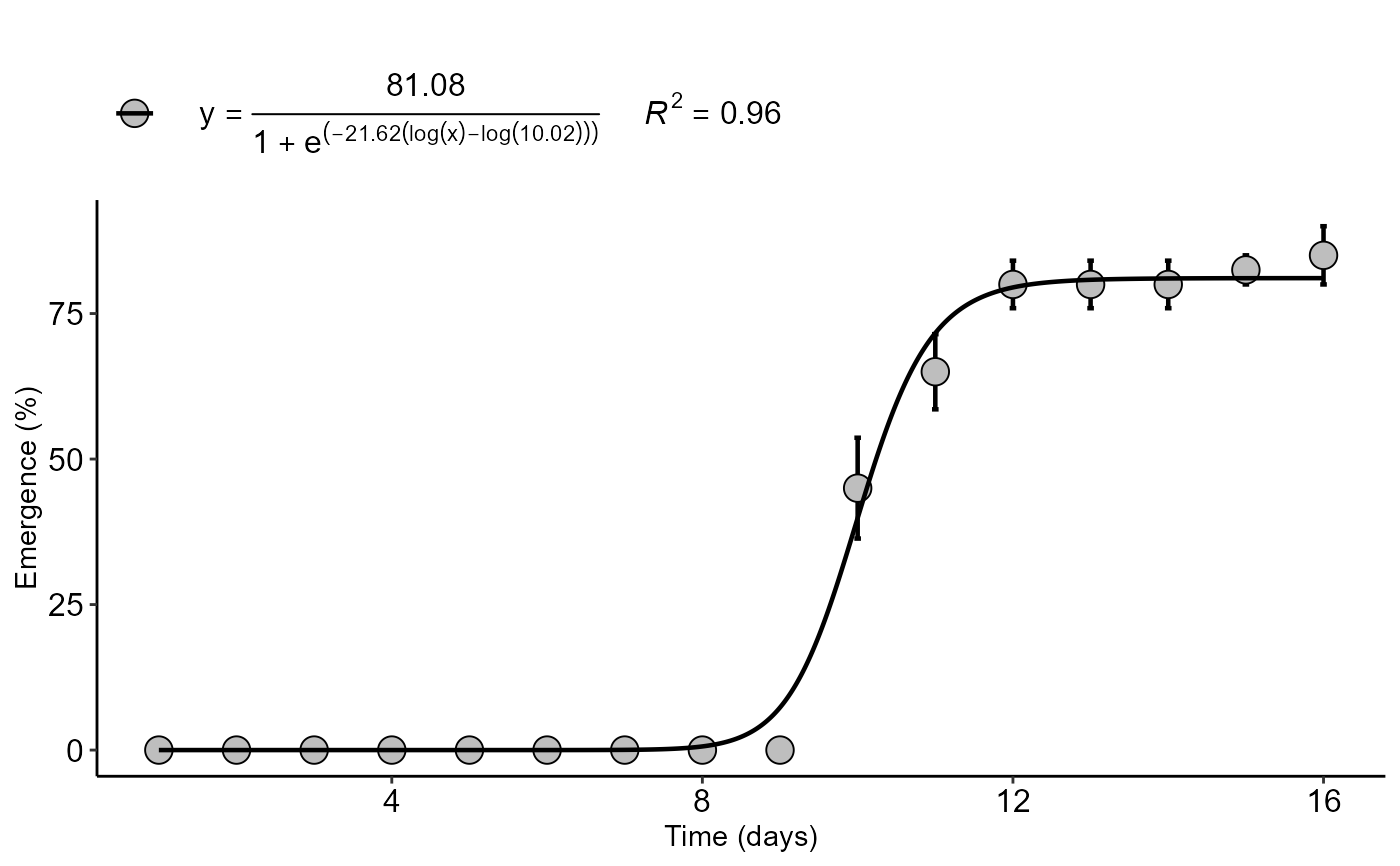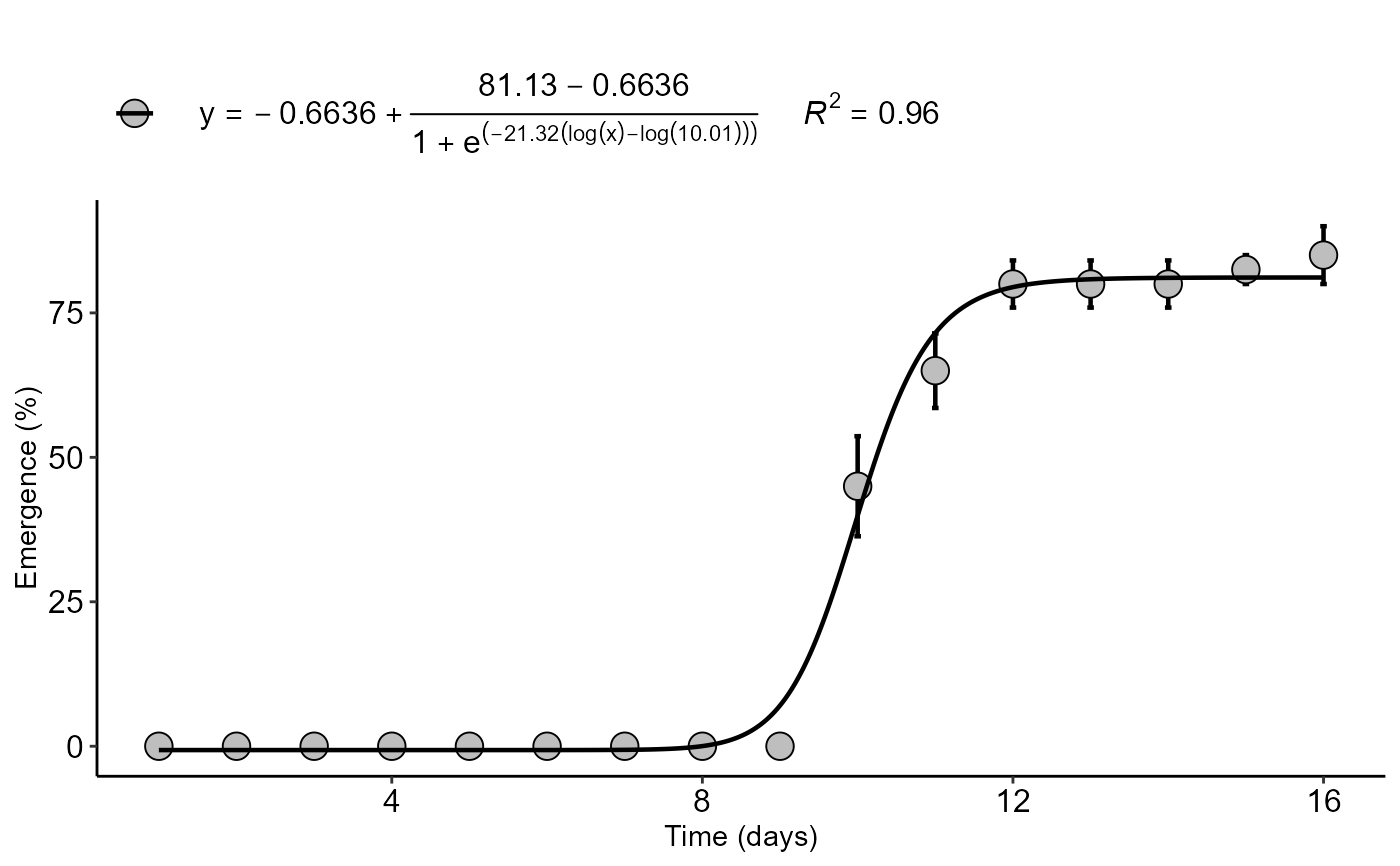Logistic regression is a very popular analysis in agrarian sciences, such as in fruit growth curves, seed germination, etc...The logistic function performs the analysis using 3 or 4 parameters of the logistic model, being imported from the LL function .3 or LL.4 of the drc package (Ritz & Ritz, 2016).

logistic(
trat,
resp,
npar = "LL.3",
error = "SE",
ylab = "Dependent",
xlab = expression("Independent"),
theme = theme_classic(),
legend.position = "top",
r2 = "all",
width.bar = NA,
scale = "none",
textsize = 12,
font.family = "sans"
)

## Arguments

trat

Numerical or complex vector with treatments

resp

Numerical vector containing the response of the experiment.

npar

Number of model parameters

error

Error bar (It can be SE - default, SD or FALSE)

ylab

Variable response name (Accepts the expression() function)

xlab

Treatments name (Accepts the expression() function)

theme

ggplot2 theme (default is theme_bw())

legend.position

Legend position (default is c(0.3,0.8))

r2

Coefficient of determination of the mean or all values (default is all)

width.bar

Bar width

scale

Sets x scale (default is none, can be "log")

textsize

Font size

font.family

Font family (default is sans)

## Value

The function allows the automatic graph and equation construction of the logistic model, provides important statistics, such as the Akaike (AIC) and Bayesian (BIC) inference criteria, coefficient of determination (r2), square root of the mean error ( RMSE).

## Details

The three-parameter log-logistic function with lower limit 0 is $$f(x) = 0 + \frac{d}{1+\exp(b(\log(x)-\log(e)))}$$ The four-parameter log-logistic function is given by the expression $$f(x) = c + \frac{d-c}{1+\exp(b(\log(x)-\log(e)))}$$ The function is symmetric about the inflection point (e).

## Author

Model imported from the drc package (Ritz et al., 2016)

Gabriel Danilo Shimizu

Leandro Simoes Azeredo Goncalves

## Examples

data("emerg")
with(emerg, logistic(time, resp,xlab="Time (days)",ylab="Emergence (%)"))
#> $Coefficients #> Estimate Std Error t value P-value #> b:(Intercept) -21.61550 3.56104842 -6.069982 8.912223e-08 ** #> d:(Intercept) 81.08478 1.73973123 46.607646 1.052258e-49 ** #> e:(Intercept) 10.01534 0.08344181 120.027785 1.852496e-74 ** #> #>$values
#>   Parameter     values
#> 1       AIC 434.790623
#> 2       BIC 443.426156
#> 3 r-squared   0.960000
#> 4      RMSE   6.789404
#>
#> $graph#> with(emerg, logistic(time, resp,npar="LL.4",xlab="Time (days)",ylab="Emergence (%)")) #>$Coefficients
#>                Estimate  Std Error     t value      P-value
#> b:(Intercept) -21.32060 3.53758836  -6.0268741 1.107976e-07 **
#> c:(Intercept)  -0.66355 1.22035166  -0.5437367 5.886374e-01 ns
#> d:(Intercept)  81.13072 1.75277913  46.2869040 5.929524e-49 **
#> e:(Intercept)  10.00733 0.08446958 118.4725590 3.826623e-73 **
#>
#> $values #> Parameter values #> 1 AIC 436.475255 #> 2 BIC 447.269671 #> 3 r-squared 0.960000 #> 4 RMSE 6.772697 #> #>$graph#>